# Quality Factor of Inductor and Capacitor

## Quality Factor of Inductor

Every inductor possesses a small resistance in addition to its inductance. The lower the value of this resistance R, the better the quality of the coil. The quality factor or the Q factor of an inductor at the operating frequency ω is defined as the ratio of reactance of the coil to its resistance.

Thus for a inductor, quality factor is expressed as,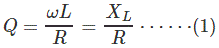Where, L is the effective inductance of the coil in Henrys and R is the effective resistance of the coil in Ohms. As the unit of both resistance and reactance is Ohm, Q is a dimensionless ratio.

The Q factor may also be defined as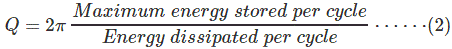Let us prove the above expression. For that let us consider a sinusoidal voltage V of frequency ω radians/seconds applied to an inductor L of effective internal resistance R as shown in Figure 1(a). Let the resulting peak current through the inductor be Im.
Then the maximum energy stored in the inductor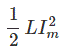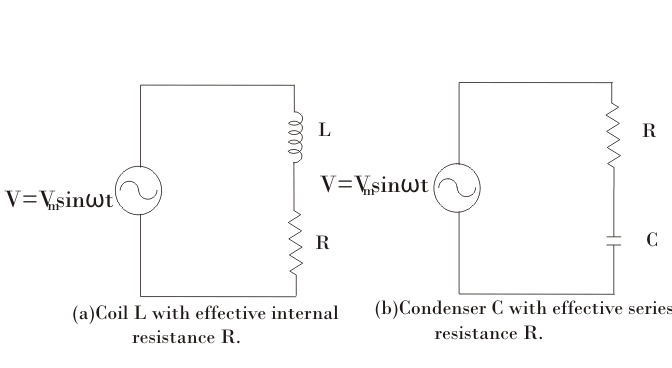Figure 1. RL and RC circuits connected to a sinusoidal voltage sources
The average power dissipated in the inductor per cycle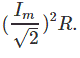Hence, the energy dissipated in the inductor per cycle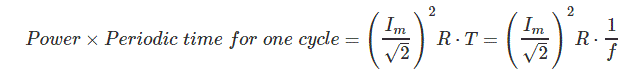Hence,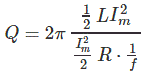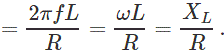## Quality Factor of a Capacitor

Figure 1(b). shows a capacitor C with small series resistance R associated within. The Q-factor or the quality factor of a capacitor at the operating frequency ω is defined as the ratio of the reactance of the capacitor to its series resistance.
Thus,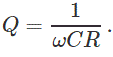In this case also, the Q is a dimensionless quantity since the unit of both reactance and resistance is the same and it is Ohm. Equation (2) giving the alternative definition of Q also holds good in this case. Thus, for the circuit of Figure 1(b), on application of a sinusoidal voltage of value V volts and frequency ω, the maximum energy stored in the capacitor.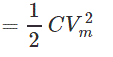Where, Vm is the maximum value of voltage across the capacitance C.
But if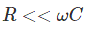then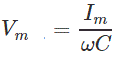Where, Im is the maximum value of current through C and R.
Hence, the maximum energy stored in capacitor C is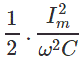Energy dissipated per cycleSo, the quality factor of capacitor is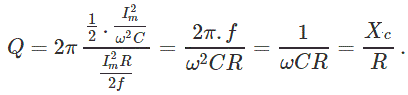Often a lossy capacitor is represented by a capacitance C with a high resistance Rp in shunt as shown in Figure 2.
Then for the capacitor of Figure 2, the maximum energy stored in the capacitor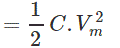Where, Vm is the maximum value of the applied voltage. The average power dissipated in resistance Rp.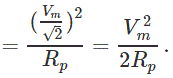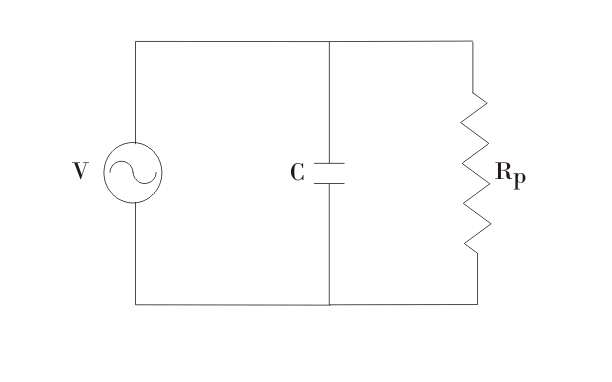Figure 2. Alternative method of representing a lossy capacitor
Energy dissipated per cycle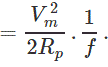Hence,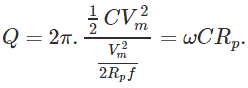Want To Learn Faster? 🎓
Get electrical articles delivered to your inbox every week.
No credit card required—it’s 100% free.